# E-PolyLearning

 1. The expression given by following equation, gives the free energy change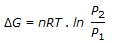a. with pressure changes at constant temperature. b. under reversible isothermal volume change. c. during heating of an ideal gas. d. during cooling of an ideal gas.

 2. The chemical potential of a component (μi) of a phase is the amount by which its capacity for doing all work, barring work of expansion is increased per unit amount of substance added for an infinitesimal addition at constant temperature and pressure. It is given by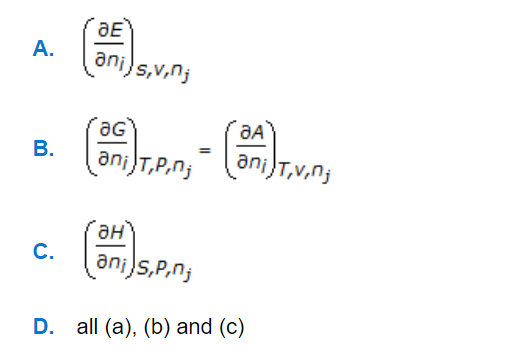a. A b. B c. C d. D
 3. Co-efficient of performance for a reversed Carnot cycle working between temperatures T₁ and T₂ (T₁ > T₂) is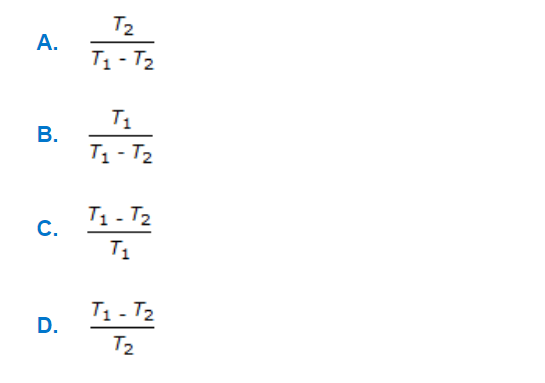a. A b. B c. C d. D
 4. Following is the mathematical expression for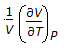a. Joule-Thomson co-efficient. b. specific heat at constant pressure (Cp). c. co-efficient of thermal expansion. d. specific heat at constant volume (CV).
 5. What is the value of maximum COP in case of absorption refrigeration, if refrigeration provided is at temperature, Tʀ(where, T₁ and T₂ are source & surrounding temperatures respectively.)?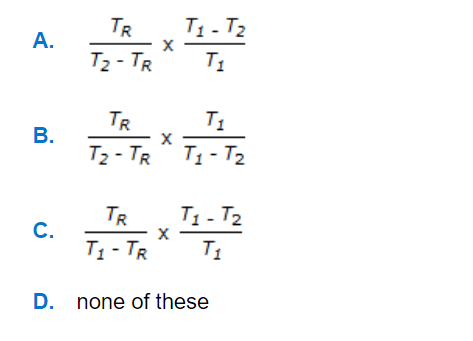a. A b. B c. C d. D